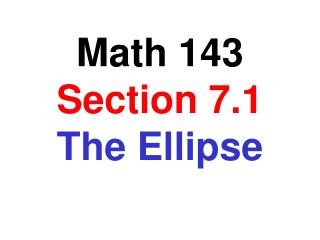DownloadDownload PresentationMath 143 Section 7.1 The Ellipse

# Math 143 Section 7.1 The Ellipse

Download Presentation## Math 143 Section 7.1 The Ellipse

- - - - - - - - - - - - - - - - - - - - - - - - - - - E N D - - - - - - - - - - - - - - - - - - - - - - - - - - -
##### Presentation Transcript

1. Math 143 Section 7.1The Ellipse

2. Ellipse An ellipse is a set of points in a plane the sum of whose distances from two fixed points, called foci, is a constant. For any point P that is on the ellipse , d2 + d1 is always the same. P d2 d1 F1 F2

3. Standard Form Equation of an Ellipse (x – h)2 (y – k)2 + = 1 a2 b2 The center of the ellipse is at the point (h, k) a is ½ the length of the horizontal axis b is ½ the length of the vertical axis Where c is the distance from the center to a focus point. c2 = a2 – b2 if a2 > b2 c2 = b2 – a2 if b2 > a2

4. Graphing an Ellipse Graph: x2 y2 4 9 + = 1 Center: (0, 0) V Minor Axis: 4 (horizontal) F Major Axis: 6 (vertical) Vertices: (0, 3) and (0, - 3) F c2 = 9 – 4 = 5 V c = Ö5 = 2.24 Foci: (0, 2.24) and (0, -2.24)

5. Graphing an Ellipse Graph: (x – 2)2 (y + 1)2 16 9 + = 1 Center: (2, -1) Major Axis: 8 (horizontal) Minor Axis: 6 (vertical) V Vertices: (6, -1) and (-2, -1) V c2 = 16 – 9 = 7 c = Ö 7 = 2.65 Foci: (4.65, -1) and (-0.65, -1)

6. Finding an Equation of an Ellipse Find the equation of the ellipse given that Vertices are (0, 4), (0, -4) Foci are (0, 3), (0, -3) V Center: (0, 0) Major Axis: 8 (vertical) b = 4 and b2 = 16 Since c = 3, and c2 = b2 – a2 9 = 16 – a2 a2 = 7 V Equation: (x – h)2 (y – k)2 a2 b2 + = 1 x2 y2 7 9 + = 1

7. Finding an Equation of an Ellipse Find the equation of the ellipse given the graph Then locate the foci of the ellipse Center: (-1, 1) V1 Major Axis: 6 (horizontal), so a = 3 Minor Axis: 2 (vertical), so b = 1 Equation: (x + 1)2 (y – 1)2 9 1 + = 1 c2 = a2 – b2 V2 c2 = 9 – 1 = 8 c = Ö8 = 2.83 (x – h)2 (y – k)2 a2 b2 + = 1 Foci are (1.83, 1), (-3.83, 1)

8. Finding an Equation of an Ellipse Find the equation of the ellipse given that Foci are (-2, 0), (2, 0) y-intercepts: -3, 3 Major Axis must be horizontal since the foci are on the major axis Center: (0, 0) c= 2 and b = 3 F F c2 = a2 – b2 4 = a2 – 9 a2 = 13 Equation: (x – h)2 (y – k)2 a2 b2 + = 1 x2 y2 13 9 + = 1

9. Converting an Equation Convert the following equation to standard form Then graph the ellipse and locate its foci 9x2 + 25y2 – 36x + 50y – 164 = 0 9(x2 – 4x + ___ ) + 25(y2 + 2y + ___) = 164 + ___ + ___ 4 1 36 25 9(x – 2)2 + 25(y + 1)2 = 225 (x – 2)2(y + 1)2 + = 1 25 9 c2 = 25 – 9 = 16 c = 4 F F Foci: (-2, -1) and (6, -1)

10. Application Problems A semielliptical archway has a height of 20 feet at its midpoint and a width of 50 feet. Can a truck that is 14 ft high and 10 ft wide drive under the archway without moving into the oncoming lane? P The real question is “What is the value of y at point P when x = 10” ? 20 10 Equation of the ellipse: x2 y2 50 + = 1 625 400 16x2 + 25y2 = 10,000 Yes, the truck will be able to drive under the archway without moving into the oncoming lane. When x = 10, 1600 + 25y2 = 10,000 25y2 = 8400 y2 = 336 y = 18.3